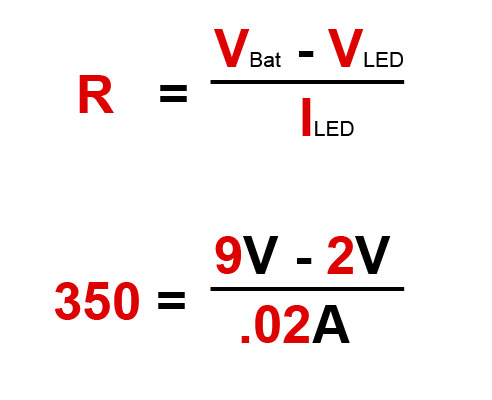# How To Determine A Resistor Size

Resistors are commonly used in electronics projects and it’s important to know which size to use.  To find the resistor value, you need to know the voltage and the amps for your LED and battery.

A standard LED generally needs a voltage of around 2V and a current of 20mA or .02A to operate correctly.  Next, you need to find out what voltage your battery is.  In this example, we will be using a 9V battery.  In order to determine the resistor size, we need to use a formula known as Ohm’s law as shown below.

Ohm’s Law –   Resistance (R) = Voltage (V) / Current (I)

• Resistance is measured in Ohms (Ω)
• Voltage is measured in volts (V)
• Current is measured in amps (A)Using Ohm’s law, you need to subtract the LED voltage from the battery voltage.  This will give you a voltage of 7 which needs to be divided by .02 amps from the LED.  This formula shows that you will need a 350 Ω resistor.

As a note, standard resistors don’t come in 350 Ω but are available in 330 Ω which will work fine.